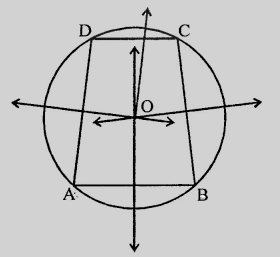# Prove that the perpendicular bisectors of the sides of a cyclic quadrilateral are concurrent.

To do:

We have to prove that the perpendicular bisectors of the sides of a cyclic quadrilateral are concurrent.

Solution:

Let $ABCD$ is a cyclic quadrilateral.We know that,

Each side of the cyclic quadrilateral is a chord of the circle and the perpendicular of a chord passes through the centre of the circle.

Therefore,

The perpendicular bisectors of each side will pass through the centre $O$.

Hence the perpendicular bisectors of the sides of a cyclic quadrilateral are concurrent.

Updated on: 10-Oct-2022

764 Views